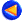### Workshop Nosevol #3

Françoise Truc : Schrödinger operators on a half-line with inverse square potentials.

We consider Schr\"odinger operators $H_\alpha=-\frac{d^2}{dx^2} + \frac{\alpha}{x^2}\$ on the half-line, with Dirichlet condition at $x=0$. We study the asymptotic behavior of the spectral density $E(H_\alpha, \lambda)$ for $\lambda \to 0$ and the $L^1\to L^\infty$ dispersive estimates associated to the evolution operator $e^{-i t H_\alpha}$. In particular we prove that for positive values of $\alpha$, the spectral density tends to zero as $\lambda\to 0$ with higher speed compared to the spectral density of Schr\"odinger operators with a short-range potential $V$. We then show that the decay rate of the evolution operator can be made arbitrarily large provided we choose $\alpha$ large enough and consider a suitable operator norm. This is a joint work with Hynek Kovarik (University of Brescia).